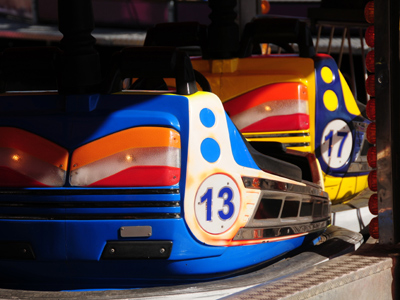Can you get ten out of ten in this quiz on times tables?

# The 13 Times Table

A knowledge of the times tables will serve you well in all levels of maths, including KS3 Maths. Most of us learn the times tables up to 12 but we thought you'd like a bit more of a challenge so we're going all the way up to 20, starting with this quiz on the 13 times table.

39, 78 and 208 are some of the answers in the 13 times table. Quite big aren't they? The number 13 isn't everybody's favourite. Writer Paul Auster said, "I've dealt with numbers all my life, of course, and after a while you begin to feel that each number has a personality of its own. A twelve is very different from a thirteen, for example. Twelve is upright, conscientious, intelligent, whereas thirteen is a loner, a shady character who won't think twice about breaking the law to get what he wants..."

Practise, practise and more practise is what it takes to master the times tables. Have a go at this quiz and see whether the number 13 is lucky or unlucky for you!

1.
What is 13 x 13
171
160
169
168
13 is the square root of 169
2.
What is 6 x 13
78
74
76
77
5 x 13 = 65
65 + 13 = 78
3.
What is 14 x 13
192
182
194
178
If you can work out 13 x 15 you then just have to subtract 13 from your answer
4.
What is 3 x 13
41
45
30
39
39 is the sum of consecutive primes: 3 + 5 + 7 + 11 + 13 = 39
5.
What is 4 x 13
52
54
50
57
There are 52 cards in a standard playing card deck - 13 in each of the 4 suits
6.
What is 10 x 13
133
128
130
129
To multiply a number by 10 simply add a zero to its end
7.
What is 8 x 13
104
103
95
107
10 x 13 = 130 and 2 x 13 = 26. 130 - 26 = 104
8.
What is 16 x 13
200
208
216
207
A tough one this. First work out 10 x 13 = 130, then calculate 6 x 13 = 78 and then just add the two answers together
9.
What is 17 x 13
216
221
230
222
It might be best to first work out 20 x 13 = 260, then subtract 3 x 13 = 39. 260 - 39 = 221
10.
What is 20 x 13
280
260
254
265
2 x 13 = 26 then add a zero to get 260
Author:  Frank Evans